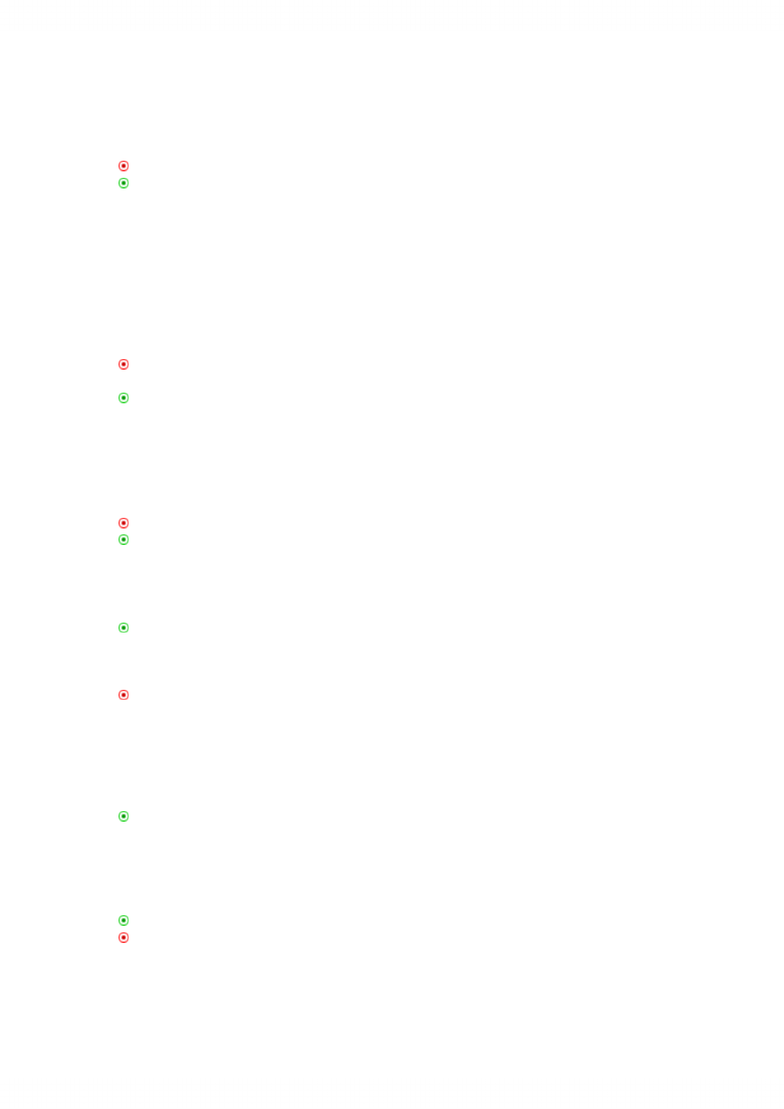Textbook Notes (290,000)
CA (170,000)
SFU (6,000)
BUS (1,000)
Chapter 2

# BUS 320 Chapter Notes - Chapter 2: Operating Cash Flow, Cash Flow, Financial Statement

Department
Course Code
BUS 320
Professor
Amir Azaran
Chapter
2

This preview shows half of the first page. to view the full 3 pages of the document.Post test
1. The accounting principle of realization is given for recording assets at their book values on
the financial statement.
a) True
b) False
The correct answer is b. The principle is supposed to be reliability. On page 29.
2. Calculate book value and market value of assets based on the information given below,
assuming that these are the only items in the Balance Sheet:
Book value Market value
Net working capital \$10,000 \$12,000
Net fixed assets 13,000 26,000
Long-term debt 13,000 17,000
Equity 10,000 11,000
a) \$13,000; \$26,000
b) \$13,000; \$28,000
c) \$23,000; \$28,000
d) \$23,000; \$38,000
e) \$24,000; \$38,000
The correct answer is d. P29
BV(asset)=NWC+NFS=10000+13000=23000
MV(asset)=12000+26000=38000
3. Assets, equity and liabilities should be stated at their market value because
a) it is an accounting rule under GAAP.
b) it is the right thing to do.
c) it yields the best measure of market value of the firm.
d) investors all over the world will demand it.
The correct answer is c. On page 29
4. Which of the following is not a short-run variable cost?
a) Property taxes
b) Wages and salaries
c) Raw material costs
d) Bonuses to executives
e) Depreciation
The correct answer is a. See the third last paragraph on p 31
5. Accounting income is different from financial cash flows because of the inclusion of non-
cash items such as ______ in the income statement.
a) depreciation and deferred taxes
b) cost of goods sold and interest expense
c) depreciation and interest expense
d) depreciation and amortization
6. The fact that costs are normally classified in accounting as either product costs or period
costs means that information in the income statement may not be all that useful for financial
managers.
a) True
b) False
The correct answer is a. On page 31
7. The definition of operating cash flow in accounting and finance are the same except for the
inclusion of ______ in the latter.
www.notesolution.com